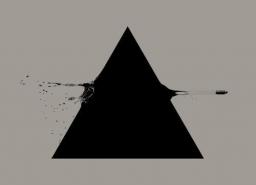# Directional 2595

Calculate the interior angles of triangle ABC using vectors. Coordinates A [2; 4] B [4; 6] C [0; -4]. Calculate directional vectors of sides, parametric and general equations of sides, parametric and general equations of lines, calculate area, calculate height.

A =  149.03 °
B =  23.198 °
C =  7.7658 °

### Step-by-step explanation:

Try calculation via our triangle calculator.Did you find an error or inaccuracy? Feel free to write us. Thank you!

Tips for related online calculators
Our vector sum calculator can add two vectors given by their magnitudes and by included angle.